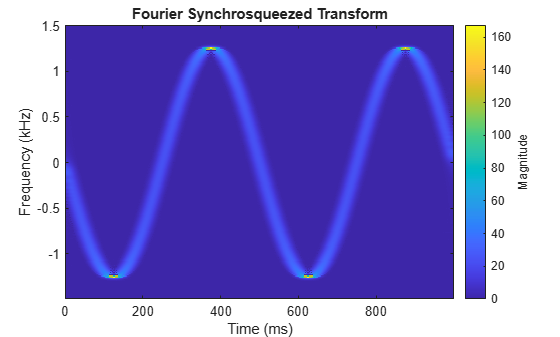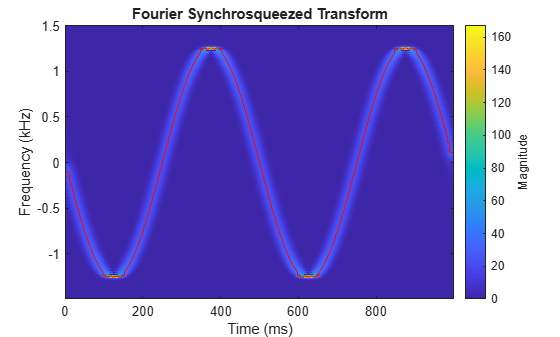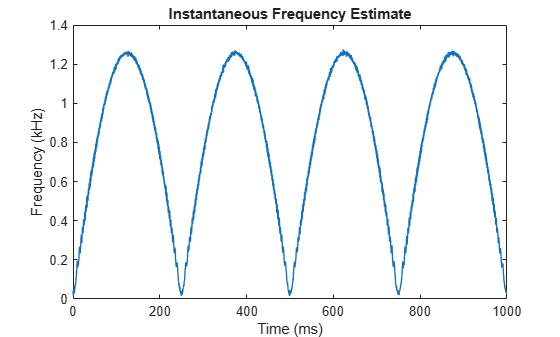Instantaneous Frequency of Complex Chirp

This example shows how to compute the instantaneous frequency of a signal using the Fourier synchrosqueezed transform.

Generate a chirp with sinusoidally varying frequency content. The signal is embedded in white Gaussian noise and sampled at 3 kHz for 1 second.

fs = 3000;
t = 0:1/fs:1-1/fs;

x = exp(2j*pi*100*cos(2*pi*2*t)) + randn(size(t))/100;

Compute and plot the Fourier synchrosqueezed transform of the signal. Display the time on the x-axis and the frequency on the y-axis.

fsst(x,fs,'yaxis')Find the instantaneous frequency of the signal by extracting the maximum-energy time-frequency ridge of the Fourier Synchrosqueezed transform.

[sst,f,tfs] = fsst(x,fs);

fridge = tfridge(sst,f);

Overlay the ridge on the transform plot. Convert time to milliseconds and frequency to kHz.

hold on
plot(t*1000,fridge/1000,'r')
hold offFor a real signal, you can find the instantaneous frequency more easily using the instfreq function. For example, display the instantaneous frequency of the real part of the complex chirp by computing the analytic signal and differentiating its phase.

ax = real(x);

instfreq(ax,fs,'Method','hilbert')